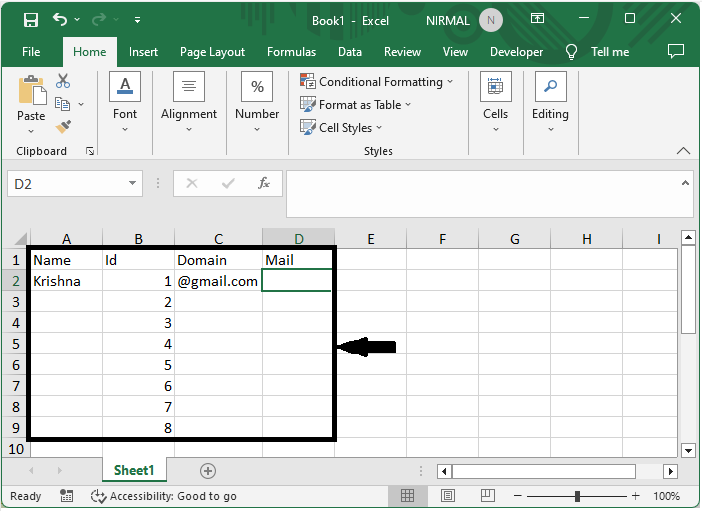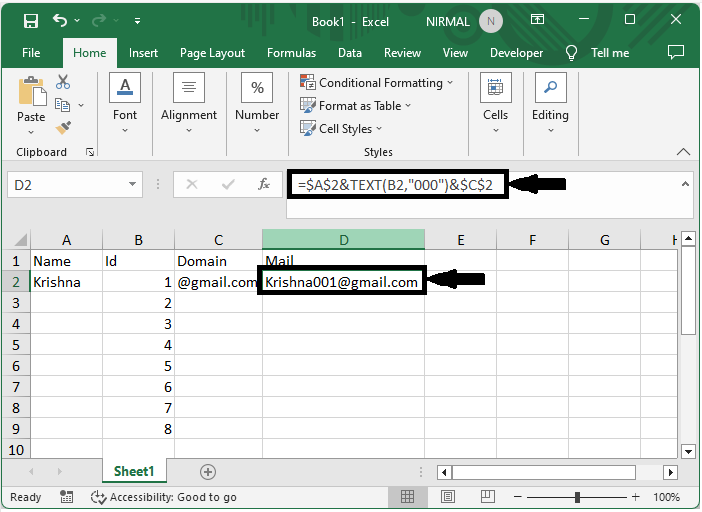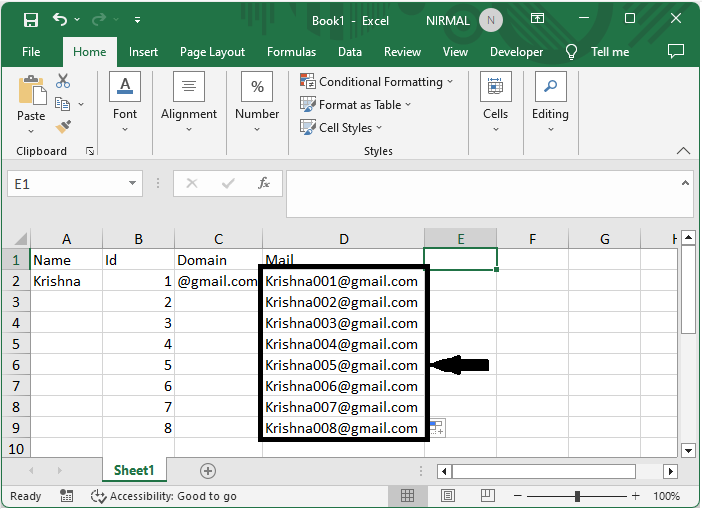# How to Create Increment Number With or Inside Text in Excel

Excel is a robust spreadsheet software that allows you to conduct numerous computations and operations on data. One common task you may find is the need to construct a succession of incrementing integers within a text string. For example, let's imagine you have a list of items that are tagged with a specific code, such as "Item001," "Item002," and so on. Instead of manually inputting each code, Excel provides an easy solution to automatically produce these incrementing numbers within the text.

You will have a strong understanding of how to use Excel's incrementing number feature within text strings at the end of this course, which will help your complete data input tasks faster and with less effort. Let's get started and discover how to insert increment numbers inside or beside text in Excel!

## Creating Increment Number With or Inside Text

Here we will use a formula to get the first value, then use the autofill handle to complete the task. So let us see a simple process to know how you can create increment numbers with or inside text in Excel.

### Step 1

Consider an Excel sheet where the data in the sheet is similar to the below image.First, click on the cell D2 and enter the formula as =$A$2&TEXT(B2,"000")&$C$2 and click enter to get the first value. In the formula, A2 is the address of text 1, B2 is a number, and C2 is text 2.

Empty cell > Formula > Enter### Step 2

Then drag down using the autofill handle to complete the task.This is how you can create an increment number with or inside the text in Excel.

## Conclusion

In this tutorial, we have used a simple example to demonstrate how you can create an increment number with or inside text in Excel to highlight a particular set of data.

Updated on: 12-Jul-2023

2K+ Views